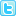ISSN: 0970-938X (Print) | 0976-1683 (Electronic)

# Biomedical Research

An International Journal of Medical Sciences

## Impacts of combining aerobic exercises with resistance training on chemerin level in obese undergraduates

Introduction: The study compared the impacts of aerobic exercises with the combination of aerobic exercises and resistance training for 16 weeks on chemerin level and blood-fat metabolism in the obese undergraduates, so as to reveal the possible mechanisms of weight loss by exercises.

Methods: 36 obese undergraduates were randomly assigned into control group, aerobic exercises group, and combining aerobic exercises with resistance training group.

Results: After 16 weeks exercise intervention, the levels of W, BMI, FM. And %F in the two intervention groups lessened much, as well as the levels of serum TG, TCH, LDL-C and chemerin reduced significantly, but the level of serum HDL-C rose much. In addition, the levels of FM, %F and serum chemerin in the combining aerobic exercises and resistance training group decreased more. With correlation analysis, we found that the level of chemerin was correlated positively with Weight (r=0.587, p=0.004), BMI (r=0.623, p=0.003), FM (r=0.722, p=0.004), %F (r=0.586, p=0.002), TG (r=0.514, p=0.013), TCH (r=0.478, p=0.032) and LDL-C (r=0.545,p=0.033) but negatively with HDL-C (r=-0.585, p=0.037).

Conclusion: Consequently, the combination of aerobic exercises and resistance training has better effects on weight loss.

Author(s): Jianyong Wang
Abstract | Full-Text | PDF

Share this